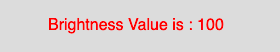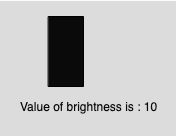Open In App
Related Articles
• Write an Interview Experience
• p5.js
• p5.js Introduction

# p5.js brightness() function

The brightness() function in p5.js is used to extract the HSB brightness value from a color or pixel array.

Syntax:

`brightness(c)`

Parameters: This function accepts single parameter c which stores the p5.Color object, color components, or CSS color.

Below programs illustrate the brightness() function in p5.js:

Example 1: This example uses brightness() function to extract the HSB brightness value from a color or pixel array.

 `function` `setup() {`` ` `    ``// Create Canvas of size 300*80``    ``createCanvas(300, 80);``}`` ` `function` `draw() {``     ` `    ``// Set background color``    ``background(220);``     ` `    ``// Initialize color mode to HSB``    ``colorMode(HSB, 255);``     ` `    ``// Initialize the parameter``    ``let c = color(0, 126, 100);``     ` `    ``// Sets 'value' to 100``    ``let value = brightness(c); ``     ` `    ``// Set the font size``    ``textSize(16);``     ` `    ``// Set the font color``    ``fill(color(``'red'``));``     ` `    ``// Display result``    ``text(``"Brightness Value is : "` `+ value, 50, 30);``}`

Output:Example 2: This example uses brightness() function to extract the HSB brightness value and fill it as gray scale integer value.

 `function setup() {`` ` `    ``// Create Canvas of size 300*180``    ``createCanvas(``300``, ``180``);``}`` ` `function draw() {``     ` `    ``// Set background color``    ``background(``220``);``     ` `    ``// Initialize color mode to HSB``    ``colorMode(HSB, ``255``);``     ` `    ``// Initialize the parameter``    ``let c = color(``0``, ``126``, ``100``);``     ` `    ``// Sets 'value' to 100``    ``let value = brightness(c); ``     ` `    ``// Fill the color ``    ``fill(value);``     ` `    ``// Create rectangle``    ``rect(``50``, ``15``, ``35``, ``70``);`` ` `    ``// Display result``    ``text(``"Value of brightness is : "` `+ value, ``22``, ``110``);``}`

Output: